## Chapter 3 Pair of Linear Equations in Two Variables R.D. Sharma Solutions for Class 10th Math Exercise 3.5

Exercise 3.5

In each of the following systems of equations determine whether the system has a unique solution, no solution or infinitely many solutions. In case there is a unique solution, find it:

1. x - 3y - 3 = 0
3x - 9y - 2 = 0

Solution

The given system of equations may be written as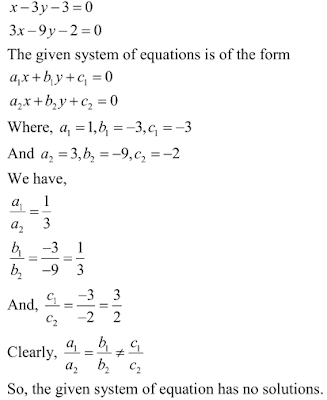2. 2x + y - 5 = 0
4x + 2y - 10 = 0

Solution

The given system of equation may be written as3. 3x - 5y = 20
6x - 10y = 40

Solution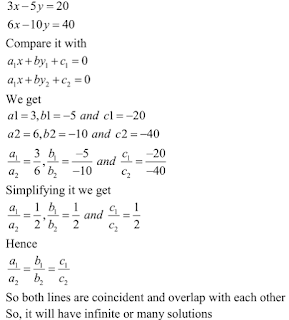4. x - 2y - 8 = 0
5x - 10y - 10 = 0

Solution

The given system of equation may be written asFind the value of k for which the following system of equations has a unique solution

5. kx + 2y - 5 = 0
3x + y - 1 = 0

Solution

The given system of equation is6. 4x + ky + 8 = 0
2x + 2y + 2 = 0

Solution7. 4x - 5y = k
2x - 3y = 12

Solution

The given system of equation isSo, the given system of equations will have a unique solution for all real values of k.

8. x + 2y = 3
5x + ky + 7 = 0

Solution

The given system of equation is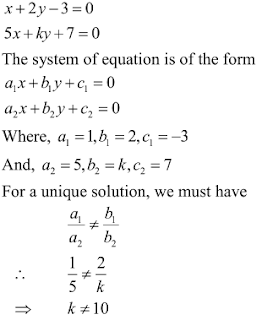So, the given system of equations will have a unique solution for all real values of k other than 10.

Find the value of k for which each of the following systems of equations have infinitely many solution: (9-19)

9. 2x + 3y - 5 = 0
6x - ky - 15 = 0

Solution

The given system of equation isHence, the given system of equations will have infinitely many solutions, if 9.

10. 4x + 5y = 3
kx + 15y = 9

Solution

The given system of equation isHence, the given system of equations will have infinitely many solutions, if k = 12.

11. kx - 2y + 6 = 0
4x + 3y + 9 = 0

Solution

The given system of equation isHence, the given system of equations will have infinitely many solutions, if k = 8/3.

12. 8x + 5y = 9
kx + 10y = 18

Solution

The given system of equation isHence, the given system of equations will have infinitely many solutions, if k = 16

13. 2x - 3y = 7
(k+2) x - (2k + 1) y - 3 (2k-1)

Solution

The given system of equation may be written as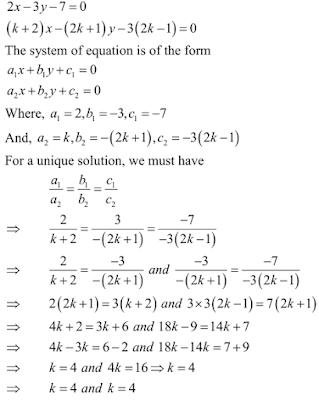Hence, the given system of equations will have infinitely many solutions, if k = 4.

14. 2x + 3y = 2
(k+2) x + (2k+1) y - (k-1)

Solution

The given system of equation may be written asHence, the given system of equations will have infinitely many solutions, if k = 4 .

15. x = (k+1) y = 4
(k+1) x + 9y - (5k+2)

Solution

The given system of equation may be written asHence, the given system of equations will have infinitely many solutions, if k = 2.

16. kx + 3y - 2k + 1
2(k+1) x + 9y - (7k+1)

Solution

The given system of equation may be written as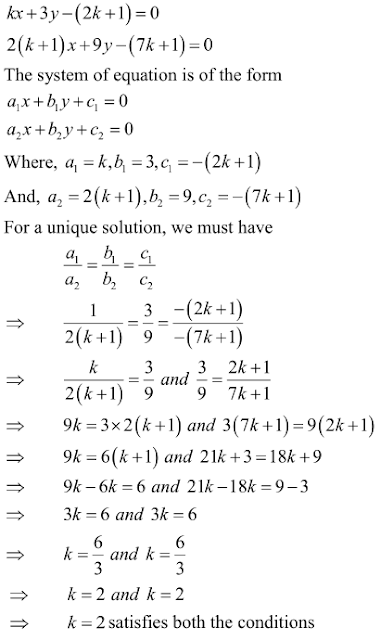17. 2x + (k-2) y = k
6x + (2k-1) y- (2k+5)

Solution

The given system of equation may be written as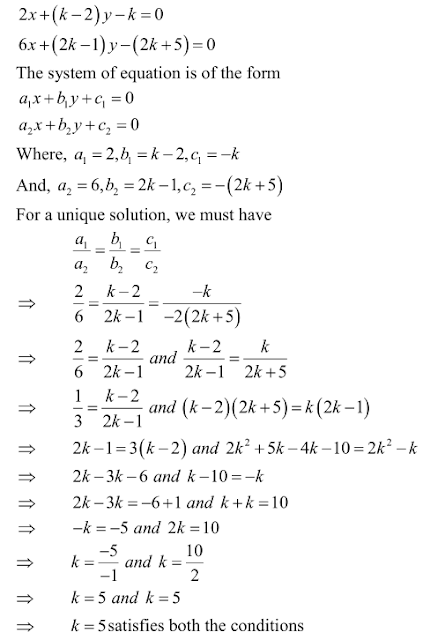Hence, the given system of equations will have infinitely many solutions, if k = 5.

18 . 2x + 3y = 7
(k+1) x + (2k-1) y - (4k+1)

Solution

The given system of equation may be written asHence, the given system of equations will have infinitely many solutions, if k = 5.

19. 2x + 3y = k
(k-1) x + (k+1) y - 3k

Solution

The given system of equation may be written asHence, the given system of equations will have infinitely many solutions, if k = 7.

Find the value of k for which the following system of equations has no solution: (20 – 25)

20. kx - 5y = 2
6x + 2y = 7

Solution

Given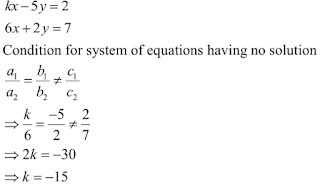21. x + 2y = 0
2x + ky - 5 = 0

Solution

The given system of equation may be written as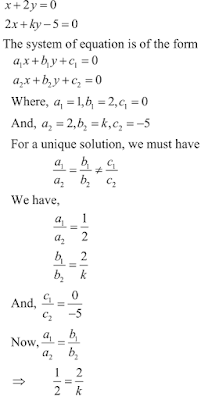Hence, the given system of equations has no solutions, when k = 4.

22. 3x - 4y + 7 = 0
kx + 3y - 5 = 0

Solution

The given system of equation may be written as23. 2x - ky + 3 = 0
3x + 2y - 1 = 0

Solution

The given system of equation may be written as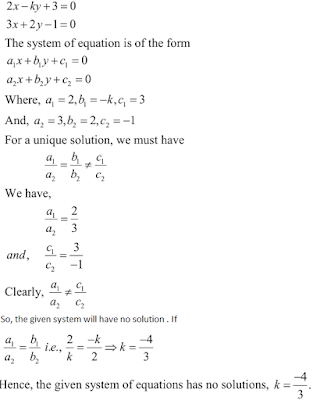24. 2x + ky = 11
5x - 7y = 5

Solution

The given system of equation is25. kx + 3y = 3
12x + ky = 6

Solution26. For what value of a, the following system of equations will be inconsistent?
4x + 6y - 11 = 0
2x + ky -7 = 0

Solution

The given system of equation may be written asHence, the given system of equation is inconsistent, when k = 3

27. For what value of a, the system of equations
ax + 3y = a - 3
12x + ay = a
will have no solution ?

Solution

The given system of equation may be written as
ax + 3y - (a - 3) = 0
12x + ay - a = 0Hence, the given system of equation will have no solution, if a = -6 .

28. Find the value of k for which the system
kx + 2y = 5
3x + y = 1
has (i) a unique solution, and (ii) no solution.

Solution

The given system of equation may be written as29. Prove that there is a value of c (≠ 0) for which the system
6x + 3y = c - 3
12x + cy = c
has infinitely many solutions. Find this value.

Solution

The given system of equation may be written as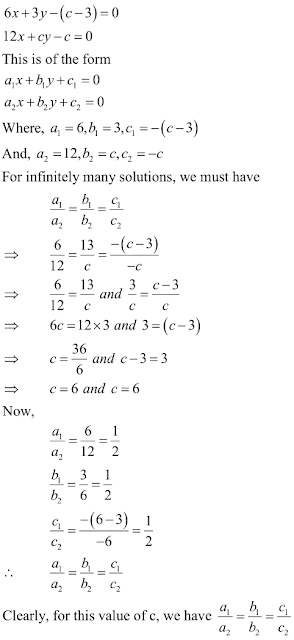30. Find the values of k for which the system
2x + ky = 1
3x – 5y = 7
will have (i) a unique solution, and (ii) no solution. Is there a value of k for which the system has infinitely many solutions ?

Solution

The given system of equation may be written as31. For what value of k, the following system of equations will represent the coincident lines ?
x + 2y + 7 = 0
2x + ky + 14 = 0

Solution

The given system of equations may be written asHence, the given system of equations will represent coincident lines, if k = 4

32. Obtain the condition for the following system of linear equations to have a unique solution
ax + by = c
lx + my = n

Solution

The given system of equations may be written asHence, am ≠ bl is the required conidition.

33. Determine the values of a and b so that the following system of linear equations have infinitely many solutions:
(2a - 1) x + 3y - 5 = 0
3x + (b - 1)y - 2 = 0

Solution

The given system of equations may be written as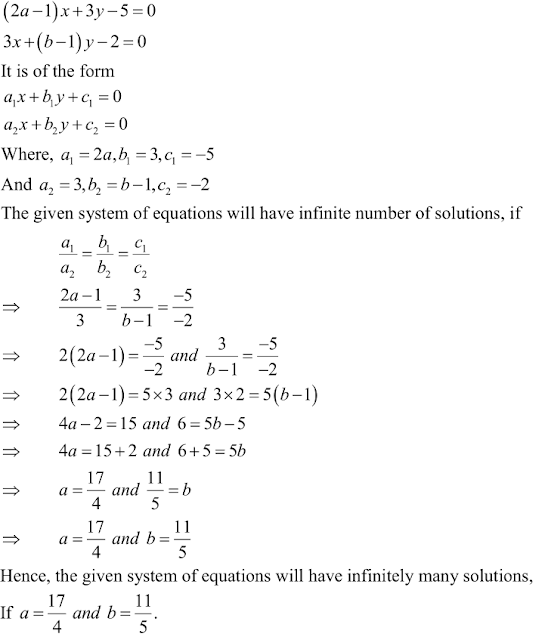34. Find the values of a and b for which the following system of linear equations has infinite number of solutions:
2x - 3y = 7
(a+b) x - (a+b-3) y = 4a + b

Solution

The given system of equations may be written asHence, the given system of equations will have infinitely many solutions, If a = -5 and b = -1 .

35. Find the values of p and q for which the following system of linear equations has infinite number of solutions:
2x - 3y = 9
(p+q) x + (2p - q) y = 3 (p+q+1)

Solution

The given system of equations may be written as36. Find the values of a and b for which the following system of equations has infinitely many solutions:
(i) 2x = 3y = 7
(a-b)x + (a+b)y = 3a+b-2

SolutionHence, the given system of equation will have infinitely many solutions,if a = 3 and b = 1/5.

(ii) 2x-(2a+5)y = 5
(2b + 1) x - 9y = 15

SolutionHence, the given system of equations will have infinitely many solutions, If a = -1 and b = 5/2.

(iii) (a-1) x + 3y = 2
6x + (1+2b) y = 6

Solution

The given system of equations is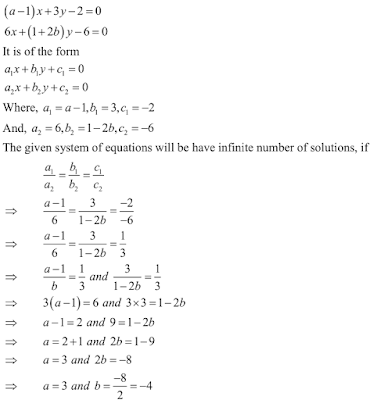Hence, the given system of equations will have infinitely many solutions, If a = 3 and b = -4.

(iv) 3x + 4y = 12
(a+b) x + 2 (a-b) y = 5a - 1

Solution

The given system of equations is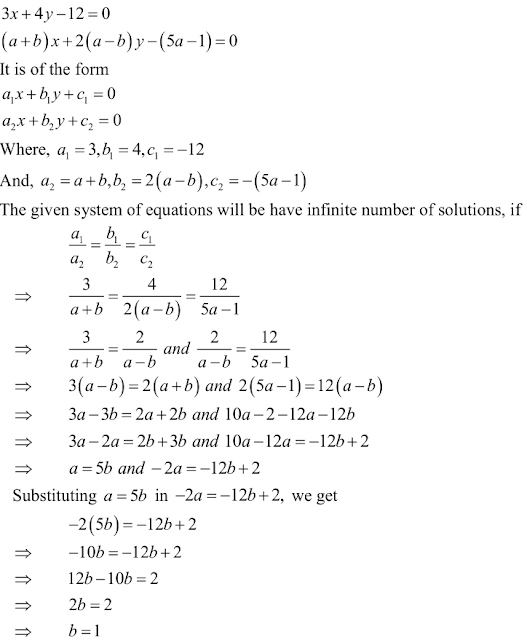Putting b = 1 in a = 5b, we get
a = 5 × 1 = 5
Hence, the given system of equations will have infinitely many solutions, If a = 5 and b = 1.

(v) 2x + 3y = 7
(a-1) x + (a+1)y = (3a-1)

Solution

The given system of equations is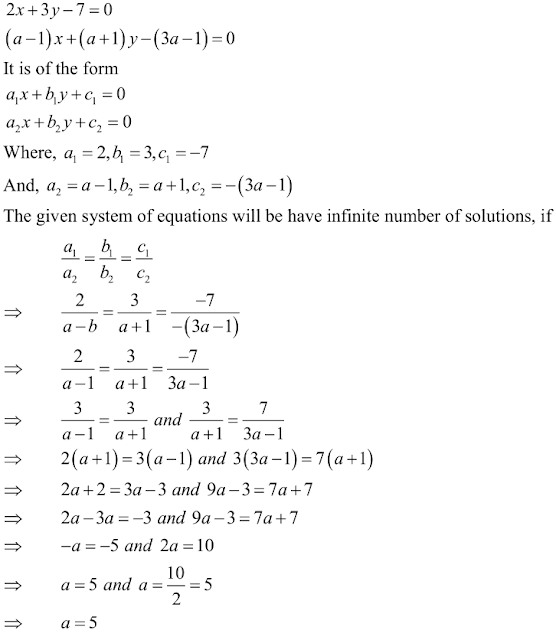Hence, the given system of equations will have infinitely many solutions, If a = 5.

(vi) 2x + 3y = 7
(a-1) x + (a+2) y = 3a

Solution

The given system of equations is